/

### Marginal Value-at-Risk (Marginal VaR)

Suppose we have a set ofrisk factors which we can characterise by an-dimensional vector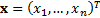. Suppose that the (active) exposures we have to these factors are characterised by another-dimensional vector,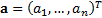. Then the aggregate exposure is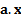.

The Value-at-Risk of the portfolio of exposures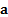at confidence level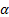,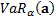, is usually defined to be the value such that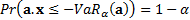.

The Marginal Value-at-Risk,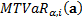, is the sensitivity of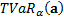to a small change in’th exposure. It is therefore: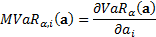Because Value-at-Risk is (first-order) homogeneous (for a continuous probability distribution) it satisfies the Euler capital allocation principle and hence: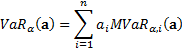If the risk factors are multivariate normally distributed then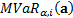can be expressed using a relatively simple formula, see here.

The Marginal Value-at-Risk is sometimes called the incremental value at risk (perhaps because a leading software vendor uses the latter terminology). More usually incremental value at risk is defined as follows, where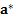is the same as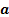except that it has 0 for its’th entry.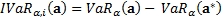Software Research Consulting Calculator Publications Examples Functions ERM Education Spreadsheets References Contact us HTML/CSS/JS Other

© 2021 - Nematrian Limited

This site uses cookies to improve and monitor its performance. Cookies may already be placed on your computer. By continuing to use this site, you are agreeing to accept these cookies which can be deleted at any time.
License Agreement etc. | Disclaimer
Desktop view | Switch to Mobile